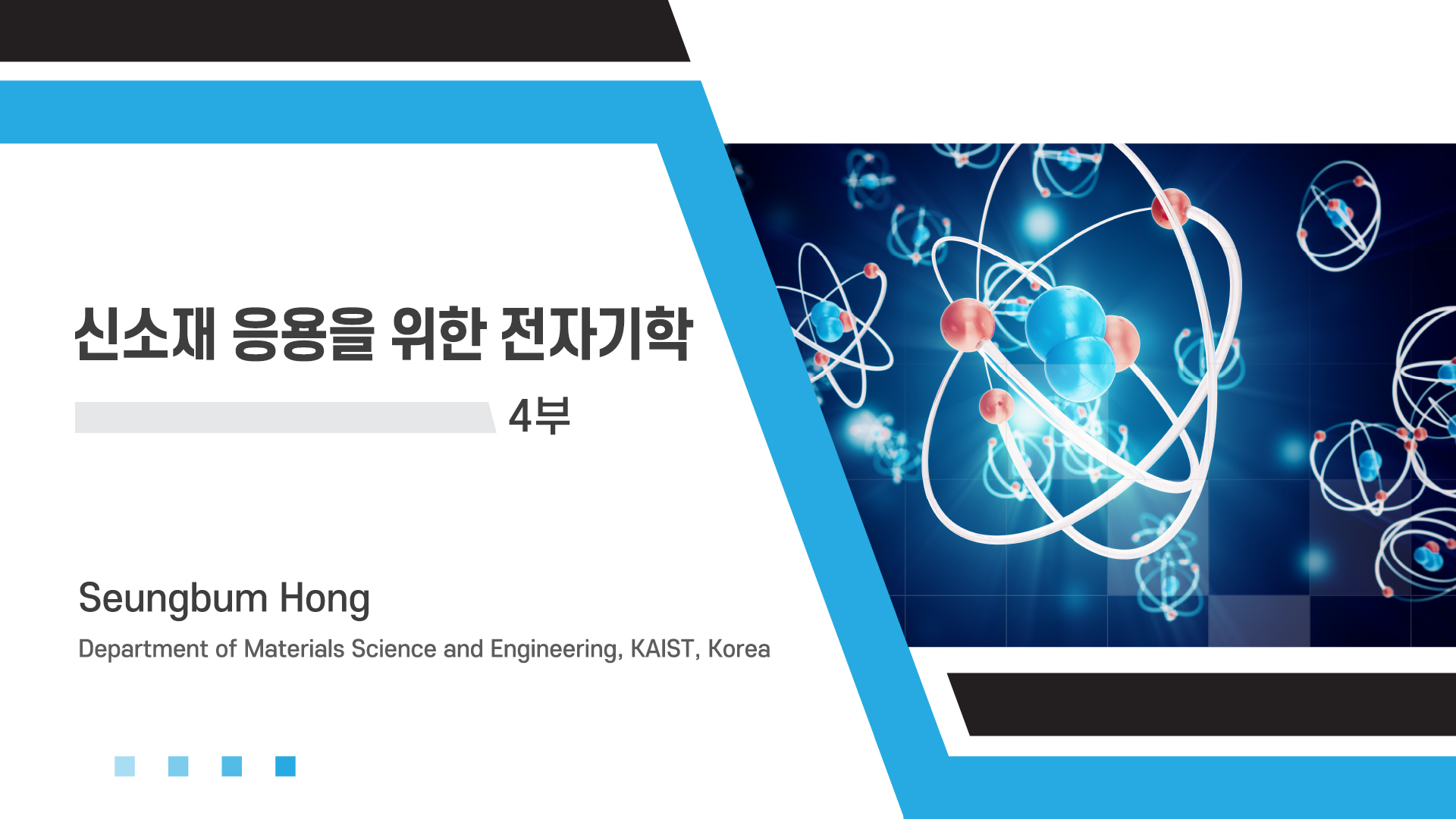## Course summary

• Type MOOC course
• Period Always open
• Learning Time Study freely
• Course approval method Automatic approval
• Certificate Issue Online
http://kaist.edwith.org/electrodynamics-04
Thumb up 12 Learner 90### Instructor Introduction

•KAIST 신소재공학과 홍승범 교수

KAIST 신소재공학과 홍승범 교수

### Lecture plan

★강의&퀴즈
1. CHAPTER 1
1. A Travelling Field - Magnetic Field
1. A Travelling Field - Electric Field
1. Speed of Light
1. Solving Maxwell's Equations
1. Quiz 1
2. CHAPTER 2
1. Waves in Free Space
1. One-Dimensional Wave Equation
1. General Wave Solution
1. Spherical Waves
1. Quiz 2
3. CHAPTER 3
1. Spherical Waves From A Point Source
1. The General Solution of Maxwell's Equations
1. An Oscillating Dipole
1. The B Field of An Oscillating Dipole
1. The E Field of An Oscillating Dipole
1. Quiz 3
4. CHAPTER 4
1. Relativistic Correction
1. Charge Moving With Constant v
1. Quiz 4
5. CHAPTER 5
1. Inductance
1. Impedance
1. Generator
1. Real Cells
1. Quiz 5
6. CHAPTER 6
1. Kirchhoff's Rules
1. Analysis of A Circuit
1. Series and Parallel Impedances
1. Energy of The Circuit